## [LATEST]\$type=sticky\$show=home\$rm=0\$va=0\$count=4\$va=0

The chapter of profit and loss is a part & parcel of every commercial activity.

This chapter has a wide-ranging importance in exams. The concepts of application percentage rule, fraction to percentage change, are applicable to profit &loss.

Cost Price : The price at which an article is brought is called its cost price, abbreviated as C.P.

Selling Price : The price at which an article is sold is called its selling price, abbreviated as S.P.

Profit or Gain = (S.P.) - (C.P.)

Loss = (C.P.) - (S.P.)

Loss or Gain is always reckoned on C.P.
Gain % = Gain x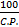Loss % = Loss xIf Profit is P% If Loss is L%
SP =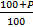x CP SP =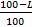x CP

CP =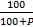x SP CP =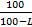x SP
Value of Profit =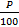x CPValue of Loss =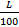x CP

Example 1: CP is 40% of SP. What is the % gain on CP?
Solution: Let the SP = Rs. 100
CP = Rs. 40
Gain = (60/40) x 100% = 150%

Example 2:The loss when SP is Rs 27.8 is equal to the gain when SP is Rs 132.2. Find CP.
Solution: CP =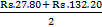= Rs. 80

Example 3:By selling 24 lemons the loss is equal to selling price of 8 lemons. Find the % Loss.
Solution:Let SP of 1 lemon = Rs. 1
Loss =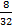x 100% = 25%

Example 4:By selling 24 lemons the gain is equal to the selling price of 8 lemons.
Find the gain %
Solution:Let SP of 1 lemon = Re. 1
Loss =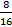x 100% = 50%

Example 5:A pretends to sell the goods at cost price but uses a weight of 960 g for 1 kg. Find his gain%.
Solution:Gain % =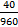x 100 = 4.17 %
(Note: we use 960 because his cost is on Rs 960 and not on Rs 1000)

Example 6:A pretends to gain 20% but also uses a weight of 800 g for 1 kg. His actual gain is.
Solution:Let CP of 1 kg = Rs. 100
therefore, SP of 800 g = Rs. 120 (Sells it at 20% gain)
Gain =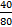x 100%Therefore, Gain is 50%

Example 7:Selling price of two articles is Rs. 910 each. Gain on one is 30%. Loss on other is 30%. Find the final position.
Solution:CP of both = Rs. 910 x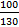+ Rs. 910 x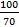= Rs. 2000
Therefore Loss = Rs. 2000 - 2. (Rs. 910) = Rs. 180

Example 8:Selling price of two articles is same. Gain on one is 20%. Loss on other 20%. On the whole the loss is ? %.
Solution:Loss = 20 x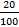% = 4%, (If SP is same.)

Example 9:If SP is Rs. 48, loss is 37%. Find the SP to gain 26%.
Solution:New S. P = 126 % of CP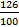x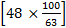= Rs. 96

Example 10:CP of 11 books = Rs. 5 SP of 10 books = Rs. 6. Find the gain percent.
Solution:Let the number of books = 110 (the LCM of 11 & 10)
CP = Rs. 50, SP = Rs. 66
Gain =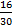x 100 = 32%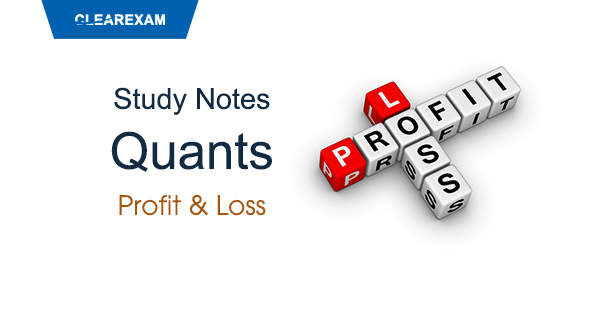Want to Know More
Please fill in the details below:

Name

ltr
item
Clear CTET - Coaching Institute for CTET, DSSSB and KVS: dsssb-quants-profit-loss-theory-part1
dsssb-quants-profit-loss-theory-part1